StatLect

# Parameter space

The concept of parameter space is found in the theory of statistical inference. In a statistical inference problem, the statistician utilizes a sample to understand from what probability distribution the sample itself has been generated. Attention is usually restricted to a well-defined set of probability distributions that could have generated the sample. When these probability distributions are put into correspondence with a set of real numbers (or real vectors), such set is called the parameter space and its elements are called parameters.## Definition

A more rigorous definition could be as follows.

Definition Let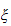be a sample (i.e., a vector of observed data). Denote by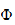the set of all probability distributions that could have generated the sample. Let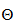be a set of real vectors. Suppose there exists a correspondence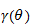that associates a subset ofto each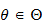. The setis called a parameter space forif and only if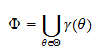The members ofare called parameters.

In other words,is a parameter space forif and only if all the probability distributions inare associated to at least one parameter, and all parameters are associated to probability distributions belonging to.

If the correspondence associates only one probability distribution to each parameter, then we have a parametric model. If there is a one-to-one correspondence between the members ofand(i.e., only one parameter is associated to each probability distribution), then the parametric model is said to be identified.

## More details

A detailed presentation of the concepts of parameter and parameter space can be found in the lecture entitled Statistical inference.

## Keep reading the glossary

Previous entry: Parameter

Next entry: Posterior probability